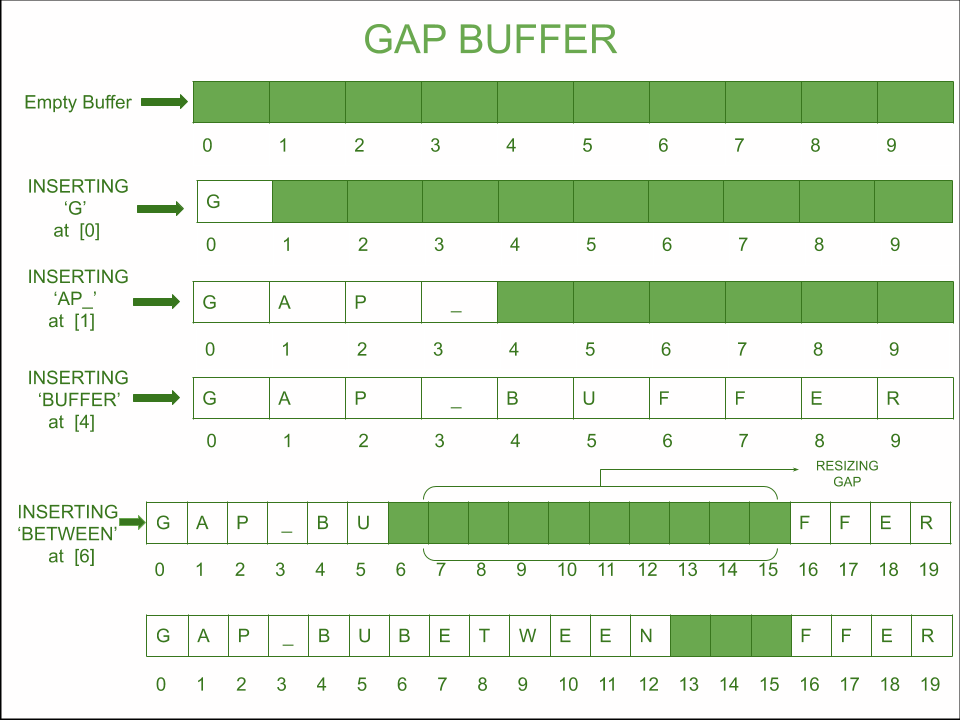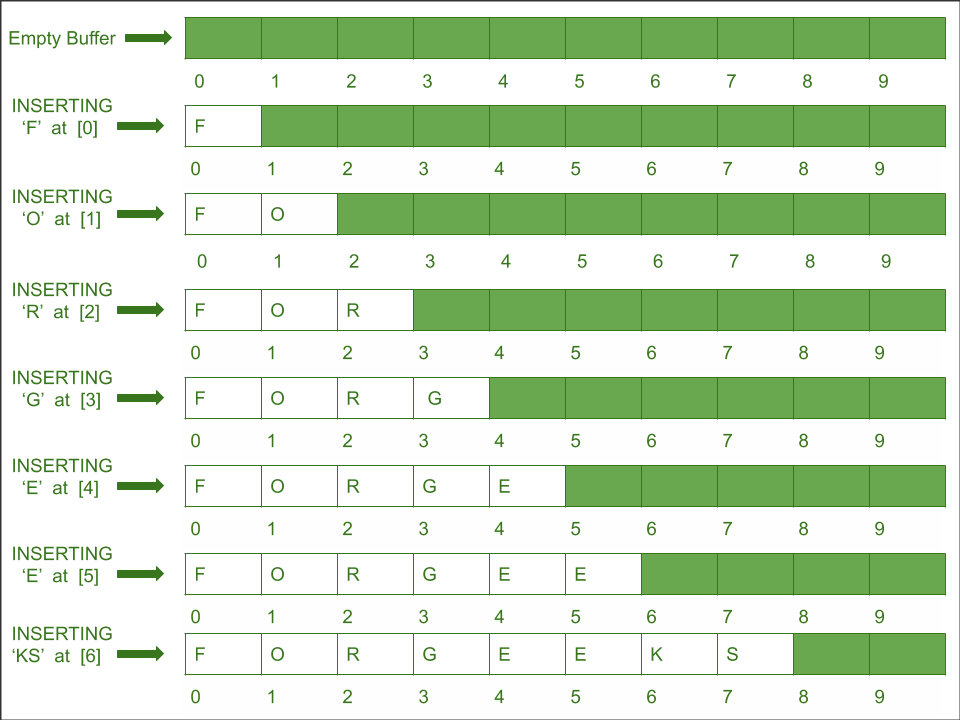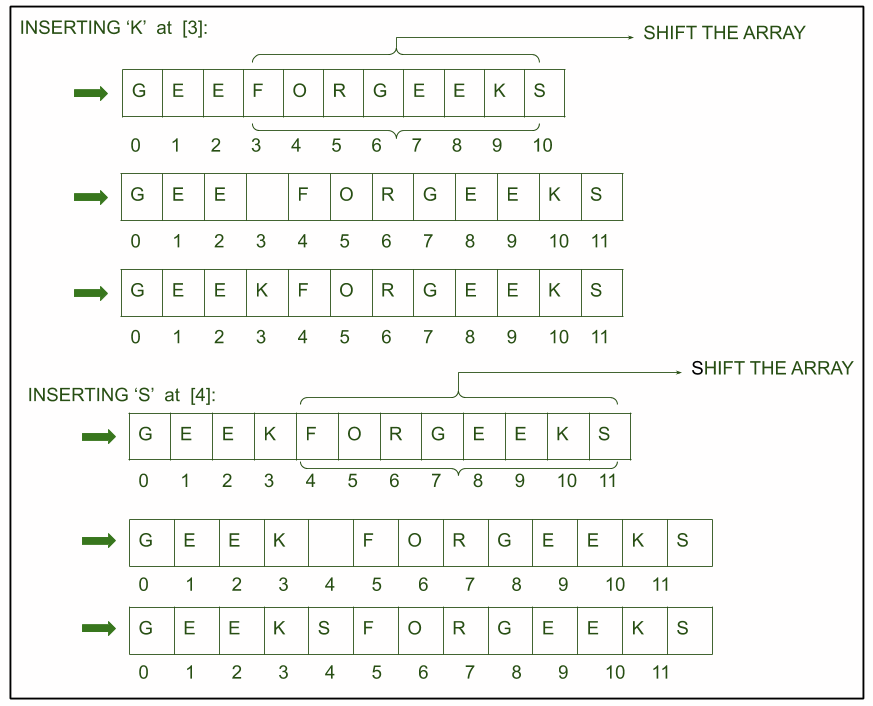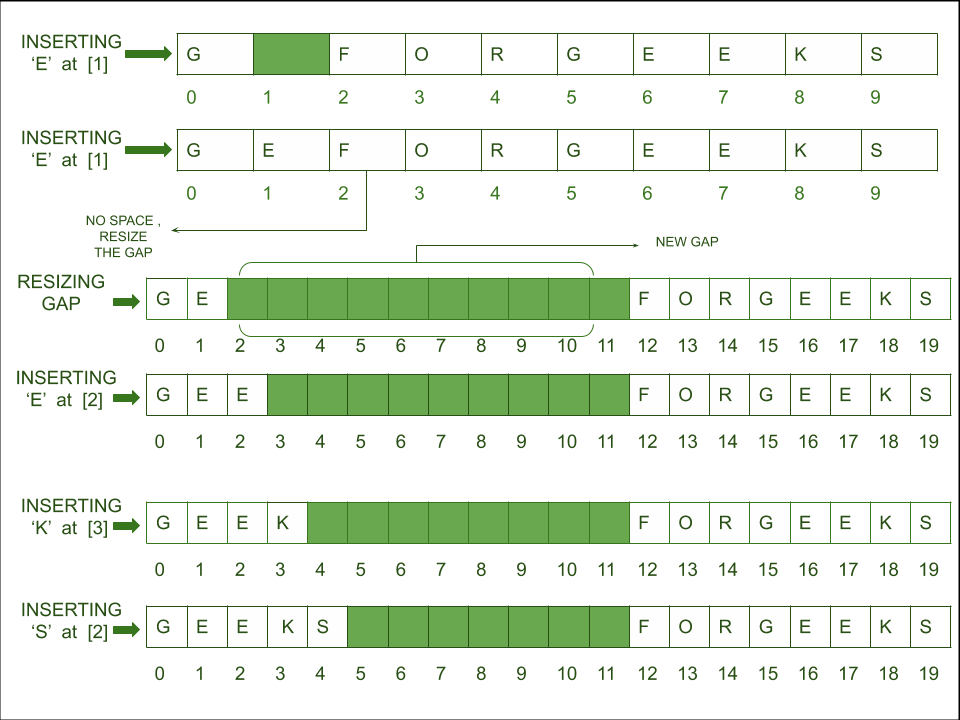# Gap Buffer Data Structure

Gap Buffer is a data structure used for editing and storing text in an efficient manner that is being currently edited. It is also similar to an array but a gap is introduced in the array for handling multiple changes at the cursor. Let’s assume a gap to be another array which contains empty spaces.Example: Consider an example with initial gap size 10, initially, array or gap are of the same size, as we insert the elements in the array similarly elements will be inserted in the gap buffer, the only difference is gap size reduces on each insert.This was the basic case to insert the character in the front. Now, whenever there is need to insert a character at certain position we will just move the gap up-to that position using left() and right() then try to insert the character.

#### Need for Gap Buffer

• Array is a data structure which stores items at contiguous memory location. However, it takes O(1) insertion at end of the array while O(n) time in front because the array will be shifted n places right, n being the length of the array.
• When it comes to text editors we need a faster data structure for insertion, modification as there are multiple changes at the cursor positions.
• In worst case array will take O(n) time for insertion or modification as shown in the example below.
• For inserting ‘GEEKS’ at the front, space is made for inserting each character by shifting the array.#### Basic operations in Gap Buffer

• insert(): It is a procedure used to insert character into the text at a given position. It first checks whether the gap is empty or not, if it finds that the gap is empty it calls procedure grow() and resizes the gap and now the element can be inserted.
• left() : It is a procedure used for moving the cursor to left and this cursor point is used as position for changes.
• right: It is a procedure used for moving the cursor to right and this cursor point is used as position for changes.
• grow: It is a procedure used when the gap size has become zero and therefore we need to resize the array by inserting a gap at desired position.• #### Gap Buffer vs Ropes

Now, although its insertion is taking O(1) time but there is another function grow() which which takes approximately O(n) time. So there might a thought that this may take the same time as the rope data structures but the cost of grow is being compensated by amortized cost of other cheaper procedures such as left(), right() and insert(). Therefore this data structure get preferences in text-editors over other such as rope as it is easy to implement.

## C++

 `// C++ program of implementation of gap buffer ` ` `  `#include   ` `using` `namespace` `std;  ` ` `  `char` `buffer;  ` `int` `gap_size = 10;  ` `int` `gap_left = 0;  ` `int` `gap_right = gap_size - gap_left-1;  ` `int` `size = 10;  ` ` `  `// Function that is used to grow the gap  ` `// at index position and return the array  ` ` `  ` `  `void` `grow(``int` `k, ``int` `position)  ` `{  ` ` `  `    ``char` `a[size];  ` ` `  `    ``// Copy characters of buffer to a[]  ` `    ``// after position  ` `    ``for` `(``int` `i = position; i < size; i++) {  ` `        ``a[i - position] = buffer[i];  ` `         `  `    ``}  ` `     `  `    ``// Insert a gap of k from index position  ` `    ``// gap is being represented by '-'  ` `    ``for` `(``int` `i = 0; i < k; i++) {  ` `        ``buffer[i + position] = ``'_'``;  ` `    ``}  ` `     `  `    ``// Reinsert the remaining array  ` `    ``for` `(``int` `i = 0; i < position + k; i++) {  ` `        ``buffer[position + k + i] = a[i];  ` `    ``}  ` ` `  `    ``size += k; ` `    ``gap_right+=k; ` `}  ` ` `  `// Function that is used to move the gap  ` `// left in the array  ` `void` `left(``int` `position)  ` `{  ` `    ``// Move the gap left character by character  ` `    ``// and the buffers  ` `    ``while` `(position < gap_left) {  ` `        ``gap_left--;  ` `        ``gap_right--;  ` `        ``buffer[gap_right+1] = buffer[gap_left]; ` `        ``buffer[gap_left]=``'_'``; ` `    ``}  ` `}  ` ` `  `// Function that is used to move the gap  ` `// right in the array  ` `void` `right(``int` `position)  ` `{  ` `    ``// Move the gap right character by character  ` `    ``// and the buffers  ` `    ``while` `(position > gap_left) {  ` `        ``gap_left++;  ` `        ``gap_right++;  ` `        ``buffer[gap_left-1] = buffer[gap_right];  ` `        ``buffer[gap_right]=``'_'``; ` `    ``}  ` `}  ` ` `  `// Function to control the movement of gap  ` `// by checking its position to the point of  ` `// insertion  ` `void` `move_cursor(``int` `position)  ` `{  ` `    ``if` `(position < gap_left) {  ` `        ``left(position);  ` `    ``}  ` `    ``else` `{  ` `        ``right(position);  ` `    ``}  ` `}  ` ` `  `// Function to insert the string to the buffer  ` `// at point position  ` `void` `insert(string input, ``int` `position)  ` `{  ` `    ``int` `len = input.length();  ` `    ``int` `i = 0;  ` ` `  `    ``// If the point is not the gap check  ` `    ``// and move the cursor to that point  ` `    ``if` `(position != gap_left) {  ` `        ``move_cursor(position);  ` `    ``}  ` ` `  `    ``// Insert characters one by one  ` `    ``while` `(i < len) {  ` `        ``// If the gap is empty grow the size  ` `        ``if` `(gap_right == gap_left) {  ` `            ``int` `k = 10;  ` `            ``grow(k, position);  ` `        ``}  ` ` `  `        ``// Insert the character in the gap and  ` `        ``// move the gap  ` `        ``buffer[gap_left] = input[i];  ` `        ``gap_left++;  ` `        ``i++;  ` `        ``position++; ` `    ``}  ` `}  ` ` `  `// Driver code  ` `int` `main()  ` `{  ` `    ``// Initializing the gap buffer with size 10  ` `    ``for` `(``int` `i = 0; i < 10; i++) {  ` `        ``buffer[i] = ``'_'``;  ` `    ``}  ` ` `  `    ``cout << ``"Initializing the gap buffer "` `         ``<< ``"with size 10"` `<< endl; ` `  `  `    ``for` `(``int` `i = 0; i < size; i++) {  ` `        ``cout << buffer[i]<<``" "``;  ` `    ``}  ` ` `  `    ``cout << endl;  ` ` `  `    ``// Inserting a string to buffer  ` `    ``string input = ``"GEEKSGEEKS"``;  ` `    ``int` `position = 0;  ` ` `  `    ``insert(input, position);  ` ` `  `    ``cout << endl;  ` `    ``cout << ``"Inserting a string to buffer"` `         ``<< ``": GEEKSGEEKS"` `<< endl;  ` `    ``cout << ``"Output: "``;  ` `    ``for` `(``int` `i = 0; i < size; i++) {  ` `        ``cout << buffer[i]<<``" "``;  ` `    ``}  ` ` `  `    ``input = ``"FOR"``;  ` `    ``position = 5;  ` ` `  `    ``insert(input, position);  ` ` `  `    ``cout << endl;  ` `    ``cout << endl;  ` `     `  `    ``cout << ``"Inserting a string to buffer"` `         ``<< ``": FOR"` `<< endl;  ` `    ``cout << ``"Output: "``;  ` `    ``for` `(``int` `i = 0; i < size; i++) {  ` `        ``cout << buffer[i]<<``" "``;  ` `    ``}  ` ` `  ` `  `    ``return` `0; ` `} `

## Java

 `// Java program of implementation of gap buffer  ` `import` `java.util.*; ` `class` `GFG  ` `{  ` `static` `char` `[]buffer = ``new` `char``[``50``];  ` `static` `int` `gap_size = ``10``;  ` `static` `int` `gap_left = ``0``;  ` `static` `int` `gap_right = gap_size - gap_left - ``1``;  ` `static` `int` `size = ``10``;  ` ` `  `// Function that is used to grow the gap  ` `// at index position and return the array  ` `static` `void` `grow(``int` `k, ``int` `position)  ` `{  ` `    ``char` `[]a = ``new` `char``[size];  ` ` `  `    ``// Copy characters of buffer to a[]  ` `    ``// after position  ` `    ``for` `(``int` `i = position; i < size; i++)  ` `    ``{  ` `        ``a[i - position] = buffer[i];  ` `    ``} ` `     `  `    ``// Insert a gap of k from index position  ` `    ``// gap is being represented by '-'  ` `    ``for` `(``int` `i = ``0``; i < k; i++)  ` `    ``{  ` `        ``buffer[i + position] = ``'_'``;  ` `    ``}  ` `     `  `    ``// Reinsert the remaining array  ` `    ``for` `(``int` `i = ``0``; i < k; i++)  ` `    ``{  ` `        ``buffer[position + k + i] = a[i];  ` `    ``}  ` ` `  `    ``size += k;  ` `    ``gap_right += k;  ` `}  ` ` `  `// Function that is used to move the gap  ` `// left in the array  ` `static` `void` `left(``int` `position)  ` `{  ` `    ``// Move the gap left character by character  ` `    ``// and the buffers  ` `    ``while` `(position < gap_left) ` `    ``{  ` `        ``gap_left--;  ` `        ``gap_right--;  ` `        ``buffer[gap_right + ``1``] = buffer[gap_left];  ` `        ``buffer[gap_left]=``'_'``;  ` `    ``}  ` `}  ` ` `  `// Function that is used to move the gap  ` `// right in the array  ` `static` `void` `right(``int` `position)  ` `{  ` `     `  `    ``// Move the gap right character by character  ` `    ``// and the buffers  ` `    ``while` `(position > gap_left) ` `    ``{  ` `        ``gap_left++;  ` `        ``gap_right++;  ` `        ``buffer[gap_left - ``1``] = buffer[gap_right];  ` `        ``buffer[gap_right]=``'_'``;  ` `    ``}  ` `}  ` ` `  `// Function to control the movement of gap  ` `// by checking its position to the point of  ` `// insertion  ` `static` `void` `move_cursor(``int` `position)  ` `{  ` `    ``if` `(position < gap_left) ` `    ``{  ` `        ``left(position);  ` `    ``}  ` `    ``else`  `    ``{  ` `        ``right(position);  ` `    ``}  ` `}  ` ` `  `// Function to insert the string to the buffer  ` `// at point position  ` `static` `void` `insert(String input, ``int` `position)  ` `{  ` `    ``int` `len = input.length();  ` `    ``int` `i = ``0``;  ` ` `  `    ``// If the point is not the gap check  ` `    ``// and move the cursor to that point  ` `    ``if` `(position != gap_left) ` `    ``{  ` `        ``move_cursor(position);  ` `    ``}  ` ` `  `    ``// Insert characters one by one  ` `    ``while` `(i < len)  ` `    ``{  ` `        ``// If the gap is empty grow the size  ` `        ``if` `(gap_right == gap_left)  ` `        ``{  ` `            ``int` `k = ``10``;  ` `            ``grow(k, position);  ` `        ``}  ` ` `  `        ``// Insert the character in the gap and  ` `        ``// move the gap  ` `        ``buffer[gap_left] = input.charAt(i);  ` `        ``gap_left++;  ` `        ``i++;  ` `        ``position++;  ` `    ``}  ` `}  ` ` `  `// Driver code  ` `public` `static` `void` `main(String[] args) ` `{ ` `    ``// Initializing the gap buffer with size 10  ` `    ``for` `(``int` `i = ``0``; i < ``10``; i++)  ` `    ``{  ` `        ``buffer[i] = ``'_'``;  ` `    ``}  ` ` `  `    ``System.out.println(``"Initializing the gap"` `+  ` `                       ``" buffer with size 10"``);  ` ` `  `    ``for` `(``int` `i = ``0``; i < size; i++)  ` `    ``{  ` `        ``System.out.print(buffer[i] + ``" "``);  ` `    ``}  ` ` `  `    ``System.out.println();  ` ` `  `    ``// Inserting a string to buffer  ` `    ``String input = ``"GEEKSGEEKS"``;  ` `    ``int` `position = ``0``;  ` ` `  `    ``insert(input, position);  ` ` `  `    ``System.out.println();  ` `    ``System.out.println(``"Inserting a string "` `+  ` `                       ``"to buffer: GEEKSGEEKS"``);  ` `    ``System.out.print(``"Output: "``);  ` `    ``for` `(``int` `i = ``0``; i < size; i++) ` `    ``{  ` `        ``System.out.print(buffer[i] + ``" "``);  ` `    ``}  ` ` `  `    ``input = ``"FOR"``;  ` `    ``position = ``5``;  ` ` `  `    ``insert(input, position);  ` ` `  `    ``System.out.println();  ` `    ``System.out.println();  ` `     `  `    ``System.out.println(``"Inserting a string"` `+  ` `                       ``" to buffer: FOR"``);  ` `    ``System.out.print(``"Output: "``);  ` `    ``for` `(``int` `i = ``0``; i < size; i++) ` `    ``{  ` `        ``System.out.print(buffer[i] + ``" "``);  ` `    ``} ` `    ``} ` `} ` ` `  `// This code is contributed by Princi Singh `

## C#

 `// C# program of implementation of gap buffer ` `using` `System; ` `     `  `class` `GFG  ` `{  ` `static` `char` `[]buffer = ``new` `char``;  ` `static` `int` `gap_size = 10;  ` `static` `int` `gap_left = 0;  ` `static` `int` `gap_right = gap_size - gap_left - 1;  ` `static` `int` `size = 10;  ` ` `  `// Function that is used to grow the gap  ` `// at index position and return the array  ` `static` `void` `grow(``int` `k, ``int` `position)  ` `{  ` `    ``char` `[]a = ``new` `char``[size];  ` ` `  `    ``// Copy characters of buffer to a[]  ` `    ``// after position  ` `    ``for` `(``int` `i = position; i < size; i++)  ` `    ``{  ` `        ``a[i - position] = buffer[i];  ` `    ``} ` `     `  `    ``// Insert a gap of k from index position  ` `    ``// gap is being represented by '-'  ` `    ``for` `(``int` `i = 0; i < k; i++)  ` `    ``{  ` `        ``buffer[i + position] = ``'_'``;  ` `    ``}  ` `     `  `    ``// Reinsert the remaining array  ` `    ``for` `(``int` `i = 0; i < k; i++)  ` `    ``{  ` `        ``buffer[position + k + i] = a[i];  ` `    ``}  ` ` `  `    ``size += k;  ` `    ``gap_right += k;  ` `}  ` ` `  `// Function that is used to move the gap  ` `// left in the array  ` `static` `void` `left(``int` `position)  ` `{  ` `    ``// Move the gap left character by character  ` `    ``// and the buffers  ` `    ``while` `(position < gap_left) ` `    ``{  ` `        ``gap_left--;  ` `        ``gap_right--;  ` `        ``buffer[gap_right + 1] = buffer[gap_left];  ` `        ``buffer[gap_left]=``'_'``;  ` `    ``}  ` `}  ` ` `  `// Function that is used to move the gap  ` `// right in the array  ` `static` `void` `right(``int` `position)  ` `{  ` `     `  `    ``// Move the gap right character by character  ` `    ``// and the buffers  ` `    ``while` `(position > gap_left) ` `    ``{  ` `        ``gap_left++;  ` `        ``gap_right++;  ` `        ``buffer[gap_left - 1] = buffer[gap_right];  ` `        ``buffer[gap_right] = ``'_'``;  ` `    ``}  ` `}  ` ` `  `// Function to control the movement of gap  ` `// by checking its position to the point of  ` `// insertion  ` `static` `void` `move_cursor(``int` `position)  ` `{  ` `    ``if` `(position < gap_left) ` `    ``{  ` `        ``left(position);  ` `    ``}  ` `    ``else` `    ``{  ` `        ``right(position);  ` `    ``}  ` `}  ` ` `  `// Function to insert the string to the buffer  ` `// at point position  ` `static` `void` `insert(String input, ``int` `position)  ` `{  ` `    ``int` `len = input.Length;  ` `    ``int` `i = 0;  ` ` `  `    ``// If the point is not the gap check  ` `    ``// and move the cursor to that point  ` `    ``if` `(position != gap_left) ` `    ``{  ` `        ``move_cursor(position);  ` `    ``}  ` ` `  `    ``// Insert characters one by one  ` `    ``while` `(i < len)  ` `    ``{  ` `        ``// If the gap is empty grow the size  ` `        ``if` `(gap_right == gap_left)  ` `        ``{  ` `            ``int` `k = 10;  ` `            ``grow(k, position);  ` `        ``}  ` ` `  `        ``// Insert the character in the gap and  ` `        ``// move the gap  ` `        ``buffer[gap_left] = input[i];  ` `        ``gap_left++;  ` `        ``i++;  ` `        ``position++;  ` `    ``}  ` `}  ` ` `  `// Driver code  ` `public` `static` `void` `Main(String[] args) ` `{ ` `    ``// Initializing the gap buffer with size 10  ` `    ``for` `(``int` `i = 0; i < 10; i++)  ` `    ``{  ` `        ``buffer[i] = ``'_'``;  ` `    ``}  ` ` `  `    ``Console.WriteLine(``"Initializing the gap"` `+  ` `                      ``" buffer with size 10"``);  ` ` `  `    ``for` `(``int` `i = 0; i < size; i++)  ` `    ``{  ` `        ``Console.Write(buffer[i] + ``" "``);  ` `    ``}  ` ` `  `    ``Console.WriteLine();  ` ` `  `    ``// Inserting a string to buffer  ` `    ``String input = ``"GEEKSGEEKS"``;  ` `    ``int` `position = 0;  ` ` `  `    ``insert(input, position);  ` ` `  `    ``Console.WriteLine();  ` `    ``Console.WriteLine(``"Inserting a string "` `+  ` `                    ``"to buffer: GEEKSGEEKS"``);  ` `    ``Console.Write(``"Output: "``);  ` `    ``for` `(``int` `i = 0; i < size; i++) ` `    ``{  ` `        ``Console.Write(buffer[i] + ``" "``);  ` `    ``}  ` ` `  `    ``input = ``"FOR"``;  ` `    ``position = 5;  ` ` `  `    ``insert(input, position);  ` ` `  `    ``Console.WriteLine();  ` `    ``Console.WriteLine();  ` `     `  `    ``Console.WriteLine(``"Inserting a string"` `+  ` `                         ``" to buffer: FOR"``);  ` `    ``Console.Write(``"Output: "``);  ` `    ``for` `(``int` `i = 0; i < size; i++) ` `    ``{  ` `        ``Console.Write(buffer[i] + ``" "``);  ` `    ``} ` `    ``} ` `} ` ` `  `// This code is contributed by 29AjayKumar `

Output:

```Initializing the gap buffer with size 10
_ _ _ _ _ _ _ _ _ _

Inserting a string to buffer: GEEKSGEEKS
Output: G E E K S G E E K S _ _ _ _ _ _ _ _ _ _

Inserting a string to buffer: FOR
Output: G E E K S F O R _ _ _ _ _ _ _ G E E K S
```

Time complexity of insert: O(1)
Time complexity of grow: O(n)

My Personal Notes arrow_drop_upCheck out this Author's contributed articles.

If you like GeeksforGeeks and would like to contribute, you can also write an article using contribute.geeksforgeeks.org or mail your article to contribute@geeksforgeeks.org. See your article appearing on the GeeksforGeeks main page and help other Geeks.

Please Improve this article if you find anything incorrect by clicking on the "Improve Article" button below.

Article Tags :

1

Please write to us at contribute@geeksforgeeks.org to report any issue with the above content.